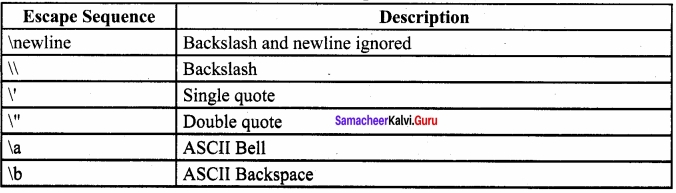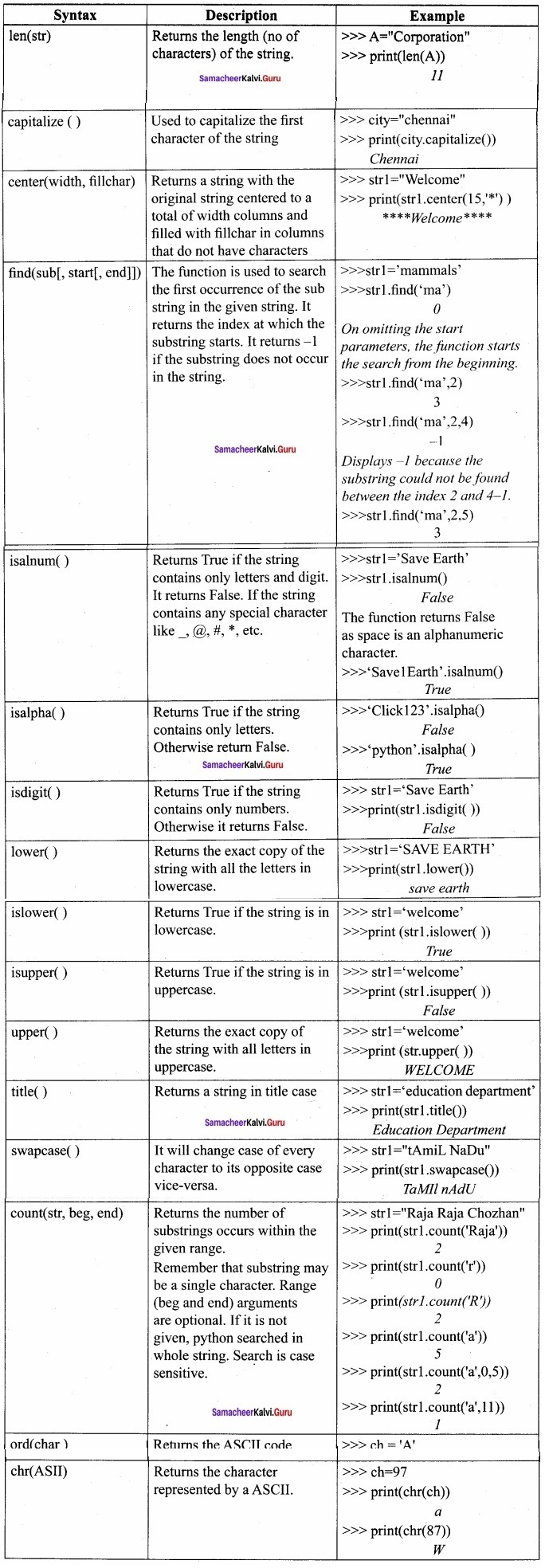# Samacheer Kalvi 12th Computer Science Solutions Chapter 8 Strings and String Manipulations

Students can Download Computer Science Chapter 8 Strings and String Manipulations Questions and Answers, Notes Pdf, Samacheer Kalvi 12th Computer Science Book Solutions Guide Pdf helps you to revise the complete Tamilnadu State Board New Syllabus and score more marks in your examinations.

## Tamilnadu Samacheer Kalvi 12th Computer Science Solutions Chapter 8 Strings and String Manipulations

### Samacheer Kalvi 12th Computer Science Strings and String Manipulations Text Book Back Questions and Answers

PART – I

12th Computer Science Chapter 8 Book Back Answers Question 1.
Which of the following is the output of the following python code?
print (str1 [:: -1])
(b) Tmlau
(c) UdanlimaT
(d) UdaNlimaT
(c) UdanlimaT

12th Computer Science Solution Book Question 2.
What will be the output of the following code?
str1= “Chennai Schools”
str1 = “_”
(a) Chennai – Schools
(b) Chenna – School
(c) Type error
(d) Chennai
(c) Type error

12th Computer Science Samacheer Kalvi Question 3.
Which of the following operator is used for concatenation?
(a) +
(b) &
(c) *
(d) =
(a) +

Samacheer Kalvi Guru 12th Computer Science Question 4.
Defining strings within triple quotes allows creating:
(a) Single line Strings
(b) Multiline Strings
(c) Double line Strings
(d) Multiple Strings
(b) Multiline Strings

Samacheer Kalvi 12th Computer Science Book Question 5.
Strings in python:
(a) Changeable
(b) Mutable
(c) Immutable
(d) Flexible
(c) Immutable

Samacheer Kalvi 12th Computer Science Book Back Answers Question 6.
Which of the following is the slicing operator?
(a) { }
(b) [ ]
(c) <>
(d) ( )
(b) [ ]

12th Computer Science Book Back Answers Question 7.
What is stride?
(a) Index value of slide operation
(b) First argument of slice operation
(c) Second argument of slice operation
(d) Third argument of slice operation
(d) Third argument of slice operation

12th Computer Science Book Answers Question 8.
Which of the following formatting character is used to print exponential notation in upper case?
(a) % e
(b) % E
(c) % g
(d) % n
(b) % E

Samacheer Kalvi Class 12 Computer Science Question 9.
Which of the following is used as placeholders or replacement fields which get replaced along with format ( ) function?
(a) { }
(b) <>
(c) ++
(d) ^^
(a) { }

Samacheer Kalvi Computer Science Book Question 10.
The subscript of a string may be:
(a) Positive
(b) Negative
(c) Both (a) and (b)
(d) Either (a) or (b)
(d) Either (a) or (b)

PART – II

Computer Science Samacheer Kalvi Question 1.
What is String?

• String is a data type in python, which is used to handle an array of characters.
• String is a sequence of Unicode characters that may be a combination of letters, numbers, or special symbols enclosed within single, double, or even triple quotes.

Example:
‘Welcome to learning Python’
“Welcome to learning Python”
“”Welcome to learning Python””

12 Computer Science Book Back Answers Question 2.
Do you modify a string in Python?
If you want to modify the string, a new string value can be assigned to the existing string variable. To define a new string value to the existing string variable. Python completely overwrites the new string on the existing string.
Example:
>>> str1=”How are you”
>>> print (str1)
How are you
>>> print (str1)

Samacheer Kalvi Guru 8th Computer Science Question 3.
How will you delete a string in Python?

• Python will not allow deleting a particular character in a string.
• We can remove the entire string variable using a del command.

Example:
>>> print (str1)
>>> del str1
The str1 ‘How about you’ will be deleted

Class 12 Computer Science Book Back Answers Question 4.
What will be the output of the following python code?
str1 = “School”
print (str1*3)
Output:
School School School

Question 5.
What is slicing?

• A slice is a substring of the main string.
• A substring can be taken from the original string by using [ ] operator and index or subscript values.
• Thus, [ ] is also known as the slicing operator.
• Using the slice operator, we have to slice one or more substrings from the main string.

Example:
strl = «THIRUKKURAL»
(i) print (str1 )
T
(ii) print (str1 [0:5])
THIRU

PART – III

Question 1.
Write a Python program to display the given pattern?
C O M P U T E R
C O M P U T E
C O M P U T
C O M P U
C O M P
C O M
C O
C
Program:
str1 = “COMPUTER”
index = len (str1)
for i in str 1:
print (str 1[: index])
index – = 1

Question 2.
Write a short about the following with a suitable example?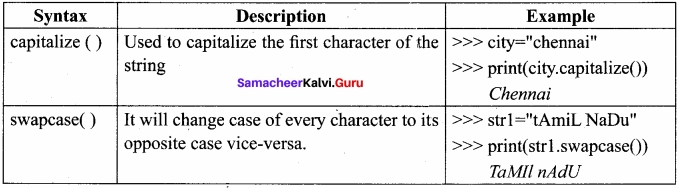Question 3.
What will be the output of the given python program?
str1 = “welcome”
str2 = “to school”
str3 = str1[: 2] str2[len(str2)-2:]
print (str3)
output:
weoo 1

Question 4.
What is the use of format( )? Give an example?
The formato function used with strings is very versatile and powerful function used for formatting strings.
The curly braces liare used as placeholders or replacement fields which get replaced along with format ()function.

Example:
numl=int (input(“Number 1: “))
num2=int (input(“Number 2: “))
print (“The, sum of {} and {} is {}”. format(numl,num2,(numl+num2)))
Output:
Number 1: 34
Number 2: 54
The sum of 34 and 54 is 88

Question 5.
Write a note about the count ( ) function in python?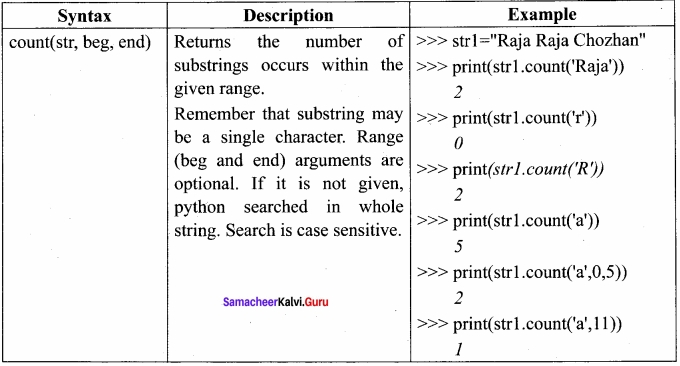PART – IV

Question 1.
Explain string operators in python with suitable examples?

• Python provides the following operators for string operations.
• These operators are useful to manipulate strings.
• They are
1. Concatenation (+):
2. Append (+ =)
3. Repeating (*)

Concatenation (+):

• Joining of two or more strings is called as Concatenation. .
• The plus (+) operator is used to concatenate strings in python.

Example:
>>> “Welcome” + “Python”
WelcomePython

Append (+ =):

• Adding more strings at the end of an existing string is known as append. *
• The operator += is used to append a new string with an existing string.

Example:
>>> str1=”Welcome to”
>>> str1+=” Learn Python”
>>> print (str1)
Welcome to Learn Python

Repeating (*):
The multiplication operator (*) is used to display a string in multiple times.

Example:
>>> strl=”‘Welcome ”
>>> print (strl *4)
Welcome Welcome Welcome Welcome

Practice Programs

Question 1.
Write a python program to find the length of a string?
str=input (“Enter a string: “)
print (len(str))
Output:
Enter a string: HELLO
5

Question 2.
Write a program to count the occurrences of each word in a given string?
def word_count(str):
counts = dict ( )
words = str.split ( ) for word in words:
if word in counts:
counts[word] +=1
else:
counts[word]=1
return counts
print (word_count (‘the quick brown fox jumps over the lazy dog.’))
Ouput:
{‘the’: 2, ‘jumps’: 1, ‘brown’: 1, ‘lazy’: 1, ‘fox’: 1, ‘over’: 1, ‘quick’: 1, ‘dog’: 1}

Question 3.
Write a program to add a prefix text to all the lines in a string?
import
text =
“‘Strings are immutable. Slice is a
substring of a main string. Stride
is a third argument in slicing operation'”
text_without_lndentation= textwrap.dedent (text)
wrapped = extwrap.fill (text_without_Indentation, width = 50)
print (textwrap.indent(wrapped, ‘*’)
print ()
Output:

• Strings are immutable. Slice is a
• substring of a main string. Stride
• is a third argument in slicing operation

Question 4.
Write a program to print integers with ‘*’ on the right of specified width?
x = 1 2 3
print (“original number: “, x)
print (“formatted number(right padding, width 6): “+” {: * < 7 d}”.format(x));
Output:
original number : 1 2 3
formatted number (right padding, width 6): 1 2 3 ***

Question 5.
Write a program to create a mirror of the given string. For example, “wel” = “lew“?
str1 = input (“Enter a string: “)
str2 = ‘ ‘
index= -1
for i in str1:
str2 += str1 [index]
index -= 1
print (“The given string = { } \n The Reversed string = { }”.format(str 1, str 2))
Output:
Enter a string: welcome
The given string = welcome
The Reversed string = emoclew

Question 6.
Write a program to removes all the occurrences of a given character in a string?
def removechar(s,c):
# find total no of occurrence of a character
counts = s.count(c)
# convert into list of characters
s = list(s)
# keep looping until counts become 0
while counts:
# remove char, from list
s.remove(c)
counts -= 1
# join remaining characters s = ” .join(s)
print(s)
s = “python programming”‘
remove char(s, ‘p’)
Output:
ython rogramming

Question 7.
Write a program to append a string to another string without using + = operator?
s1 = input (“Enter the first string: “)
s2 = input (“Enter the second string: “)
print (‘concatenated strings =’,” ” ,join ([s1, s2]))
Output:
Enter the first string: Tamil

Question 8.
Write a program to swap two strings?
print (“Enter Y for exit.”)
string1 = input(“Enter first string: “)
if string1 = = ‘x’:
exit();
else:
string2 = input (“Enter second string : “)
print (” \n Both strings before swap : “)
print (“First string = “, string1)
print (” Second string = “, string2)
temp = string1
string1 = string2
string2 = temp
print (“\n Both strings after swap: “)
print (“First string = “, string1)
print (” Second string = “, string2)
Output:
Enter ‘x’ for exit
Enter first string: code
Enter second string: python
Both strings before swap:
First string = code
Second string = python
Both strings after swap:
First string = python
Second string = code

Question 9.
Write a program to replace a string with another string without using replace ( )?
s1 = input (“Enter the string to be replaced: “)
s2 = input (“Enter the string to replace with “)
s1 = s2
print (“Replaced string is “, s1)
Output:
Enter the string to be replaced: Computer
Enter the string to replace with: repcomputer
Replaced string is repcomputer

Question 10.
Write a program to count the number of characters, words and lines in a given string?
string = input (“Enter string:”)
char = 0
word = 0
line = 0
for i in string:
char = char + 1
if (i = = “):
word = word + 1
elif (i = = ‘ \n’):
line = line +1
print (“Number of words in the string: “)
print (word)
print (“Number of characters in the string: “)
print (char)
print (“Number of lines in the string: “)
print (line)
Output:
Enter string: welcome to learning python
Number of words in the string : 4
Number of characters in the string : 26
Number of lines in the string : 1

### Samacheer Kalvi 12th Computer Science Strings and String Manipulations Additional Questions and Answers

PART – 1

Question 1.
Which of the following is used to handle an array of characters in python?
a) Functions
b) Composition
c) String
d) Arguments
c) String

Question 2.
Strings which contains double quotes should be defined with …………………….. quotes
(a) Single
(b) Double
(c) Triple
(d) All the these
(c) Triple

Question 3.
Which of the following allows the creation of multiline strings
a) ‘ ‘
b) ” ”
c) “”” “””
d) None of these
c) “”” “””

Question 4.
In strings, the negative index assigned from the last character to the first character in reverse order begins with …………………………
(a) 0
(b) 1
(c) -1
(d) -2
(c) -1

Question 5.
How will you modify the string?
(a) A new string value can be assigned to the existing string variable
(b) Updating the string character by character
(a) A new string value can be assigned to the existing string variable

Question 6.
The positive subscript always starts with
a) 0
b) 1
c) -1
d) 0.1
a) 0

Question 7.
Which command is used to remove the entire string variable?
(a) Remove
(b) Del
(c) Delete
(d) Strike
(b) Del

Question 8.
The joining of two or more strings is called…………………………..
(a) Append
(b) Repeating
(c) Concatenation
(d) Strike
(c) Concatenation

Question 9.
…………………. are immutable in python.
a) Characters
b) Strings
c) Numbers
d) Functions
b) Strings

Question 10.
Find the wrongly matched pair from the following.
(a) Append ⇒ + =
(b) Concate ⇒ +
(c) Repeat ⇒ /
(d) Slice ⇒ [ ]
(c) Repeat ⇒ /

Question 11.
Which operator is used to append a new string with an existing string?
(a) +
(b) + =
(c) *
(d) * =
(b) + =

Question 12.
The multiplication operator is also called………………………….
(a) Append
(b) Concatenate
(c) Repeat
(d) Slice
(c) Repeat

Question 13.
Which is used to display a string multiple times?
(a) Repeating
(b) *
(c) Multiplication operator
(d) All the above
(d) All the above

Question 14.
…………………………. is a substring of the main string.
Slice

Question 15.
The formatting operator is used to replacing parts of strings with the data stored in variables.
a) #
b) %
c) ::
d) ,
b) %

Question 16.
Find the wrong statement from the following.
(I) Slice a single character from a string
(II) Slice a substring
(III) Slice a substring without specifying the beginning index
(IV) Slice a substring without specifying the end index

(a) (I), (II)
(b) (II), (III), (IV)
(c) All are wrong
(d) All are correct
(d) All are correct

Question 17.
The default value of stride is …………………………
(a) 0
(b) 1
(c) n
(d) n – 1
(b) 1

Question 18.
If the stride is negative, then it will prints
(a) Third character
(b) the Third word
(c) Full string
(d) Reverse order
(d) Reverse order

Question 19.
…………………………. is the formatting character for signed decimal integer.
(a) %d or %i
(b) %d and %i
(c) %d %u
(d) %i &u
(a) %d or %i

Question 20.
…………………….. is the formatting character for short numbers in floating-point or exponential notation.
% g or % G

Question 21.
Back Slash

Question 22.
Find the wrong match
(a) Backslash – \b
(b) Backslash – //
(c) Carriage return – \r
(d) Line feed – \n
(b) Backslash – //

Question 23.
Which function returns the length of the string?
(a) str len( )
(b) len(str)
(c) length( )
(d) strlength( )
(b) len(str)

Question 24.
The function isalnum( ) returns ………………………. when it contains special characters.
False

Question 25.
How many membership operators are there?
(a) 2
(b) 3
(c) 4
(d) 5
(a) 2

Question 26.
……………………. is the membership operator.
(a) is
(b) at
(c) to
(d) in
(d) in

Question 27.
……………………. function is a powerful function used for formatting strings.
Format ( )

Question 28.
The ……………………. and ………………………. operators can be used with strings to determine whether a string is present another string.
In, Not in

PART – II

Question 1.
Fill the Table with appropriate values.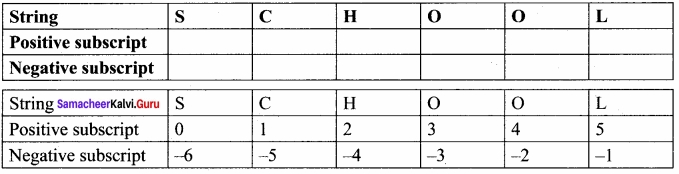Question 2.
Find the output?
Program
str1 = ‘ * ‘
i=1
while i<=5: print (str1*i)
i+=1
Output
*
* *
* * *
* * * *
* * * * *

PART – III

Question 1.
Write a note on replacing function?
The replace function replaces all occurrences of char 1 with char 2.
Example
>>> str1 =”How are you”
>>> print (str1)
How are you
>>>print (str1.replace(“o”, “e”))
Hew are yeu

Question 2.
Write a note on the function center ().
Function :center ()
Syntax:
center (width, fillchar)

Description:
Returns a string with the original String centered to a total of width columns and filled with fillchar in columns that do not have characters.

Example:
>>>strl=”Welcome”
>>>print(strl center(15,’*’))
**** Welcome****

Question 3.
Give any 6 formatting characters with their usage?
Formatting characters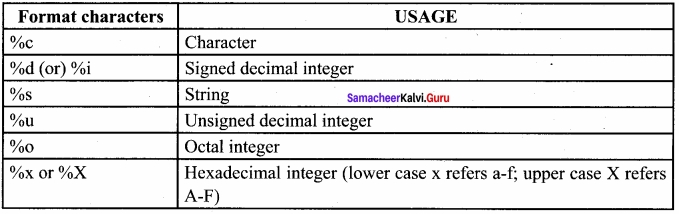Question 4.
Write any 6 escape sequences with their description?